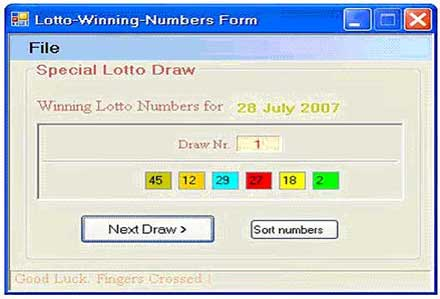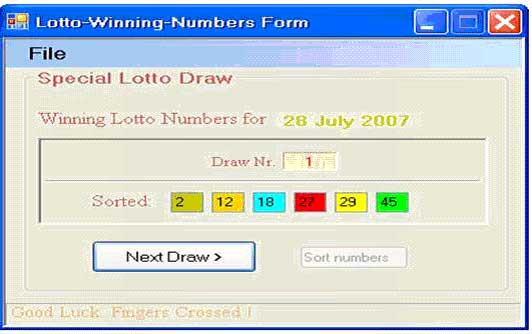# Lotto Program in C#

## IntroductionFigure 1.

This is a small Lotto-Program which can help you fill your lotto ticket. It is written in C#.NET 2.0 by using Visual Studio 2005.

If you run it will display the 6 Lotto numbers in the 6 TextBoxes for each draw. Every time you click "Next Draw" button the numbers are displayed in unsorted order but by clicking the "Sort numbers" button they will be then displayed sorted in the TextBoxes.

##Figure 2.  How it works and the code

As you can see in the code if the program runs there is an algorithm that determines which 6 random numbers are stored in an array beginning from 1 to 49. The 6 random numbers are in unsorted order in Textboxes because if you watch the "Lotto Draw Show" on the telly the winning Lotto numbers appear at the beginning unsorted on the screen. And then the speaker repeats the winning Lotto numbers but this time in sorted order. So the "Sort numbers" button does the same job as the speaker.

And finally the current date and the number of each draw are displayed, too.
It doesn't pick the winning numbers all the time, it just shows you which numbers the most time are selected randomly. The algorithm (got from internet years ago) is not the best for a professional lotto-application but I think it is good just for programming and fun.

private void frmLotto_Load(object sender, EventArgs e)

{

int c = 1000;

int[] numb = new int[c];

DateTime dt = new DateTime();

dt = DateTime.Now;

Random rnd = new Random(dt.Millisecond);

for (int i = 1; i < c; i++)

{

numb[i] = rnd.Next(1, 50);

}

Array.Sort(numb);

int k = 0;

int j = 0;

for (int i = 0; i < c - 1; i++)

{

if (numb[i] == numb[i + 1])

{

result[k] = numb[i];

lottonumbers[k] = j += 1;

}

else

{

k += 1;

j = 0;

}

}

//sort all of the numbers

Array.Sort(lottonumbers, result);

// first display numbers unsorted

fnDisplay6NumbersUnsorted();

//get the date of today

DateTime dt1 = DateTime.Today;

//display date

this.labelDaytime.Text = dt1.ToLongDateString();

//display which draw number

this.label4.Text = n_draw.ToString();

//don´t show text "sorted:"

this.labelSort.Visible = false;

this.pbNextDraw.Focus();

}

private void fnDisplay6NumbersUnsorted()

{

this.textBox1.Text = result.ToString();

this.textBox2.Text = result.ToString();

this.textBox3.Text = result.ToString();

this.textBox4.Text = result.ToString();

this.textBox5.Text = result.ToString();

this.textBox6.Text = result.ToString();

}

The algorithm starts by the PCs millisecond and then the numbers going through between 1-49 are sorted in an array by number from lowest to highest. In the second loop: how many times a number appears in the array that number is then stored sorted in another array. (Number appearing the least to number appearing the most) Finally the last 6 numbers(the six most picked) of the array are displayed.

Here is the code snippet for the button "Sort numbers" if clicked.

private void pbSort_Click(object sender, EventArgs e)

{

//call the methode to sort

//disable the sort pushbutton

this.pbSort.Enabled = false;

//enable the sort Label "sorted"

this.labelSort.Visible = true;

this.pbNextDraw.Focus();

}

{

//temporary array

int[] nr = new int;

//put all 6 numbers from TextBoxes into one array

nr = Convert.ToInt16(this.textBox1.Text);

nr = Convert.ToInt16(this.textBox2.Text);

nr = Convert.ToInt16(this.textBox3.Text);

nr = Convert.ToInt16(this.textBox4.Text);

nr = Convert.ToInt16(this.textBox5.Text);

nr = Convert.ToInt16(this.textBox6.Text);

//sort the new temporary array nr

Array.Sort(nr);

//now assign the 6 numbers from the new array nr to all TextBoxes and display

this.textBox1.Text = nr.ToString();

this.textBox2.Text = nr.ToString();

this.textBox3.Text = nr.ToString();

this.textBox4.Text = nr.ToString();

this.textBox5.Text = nr.ToString();

this.textBox6.Text = nr.ToString();

}

Conclusion:

Please, don't consider the application to be as a professional lotto-application, but treat it as a C#-lotto-demo-program, as starting point for your own software. However the application may be useful for many personal reasons.

I hope you liked the article, happy coding!